# Financial literacy resources

## Lessons

Explore and download a wide range of free financial literacy lessons for students in middle and high school.
Made for use with TI-84 Plus CE or TI-Nspire™ CX II graphing calculators.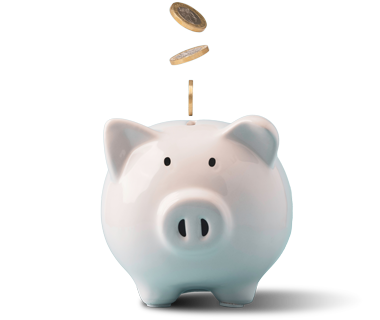## Loans: Days Between Dates

By determining the number of days between dates, students will find the amount due on a loan at the end of a term.

## Compound Interest

Understand how interest from a previous period is added to the current principal and how the new total is used as the basis for calculating the interest.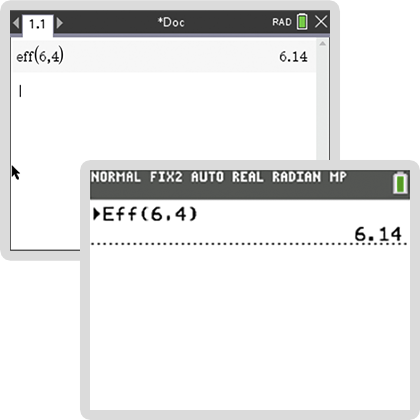## Effective Annual Rate and Nominal Rate

Students use the effective annual rate calculation to compare the nominal rates of various financial instruments.

## Continuous Compounding

Learn how to deal with financial computations where the interest is compounded continuously. Depending on the length of each compounding period, students will determine the number of compounding periods.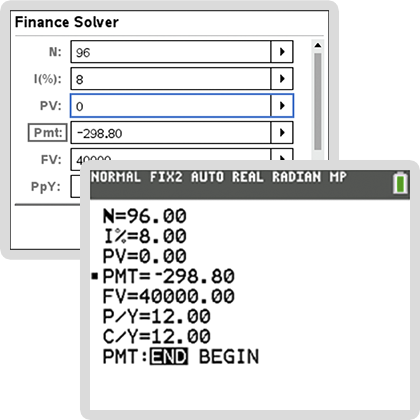## Future Value of an Ordinary Annuity andSinking Funds

Students carry out financial computations involving annuity and the future value of annuities while also considering computations involving sinking funds.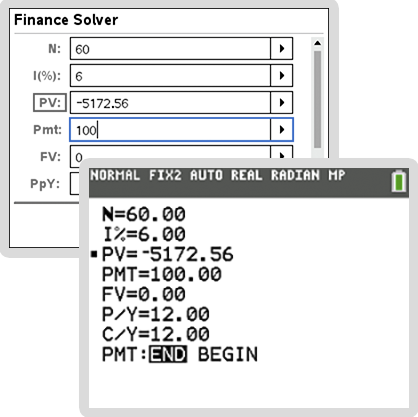## Present Value of an Ordinary Annuity

Find out how much money should be deposited today so that a fixed amount can be drawn at regular intervals. These calculations involve determination of the present value of annuity.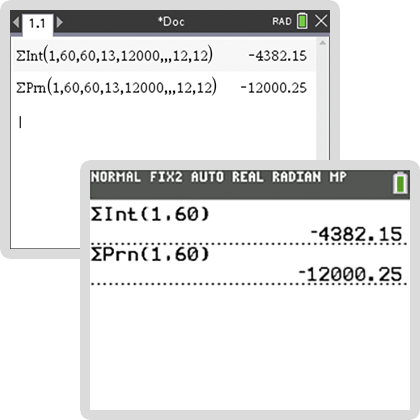## Loans and Mortgages

In this activity, students perform financial computations involving loans, their repayment, and mortgages used as security for the repayment of a loan.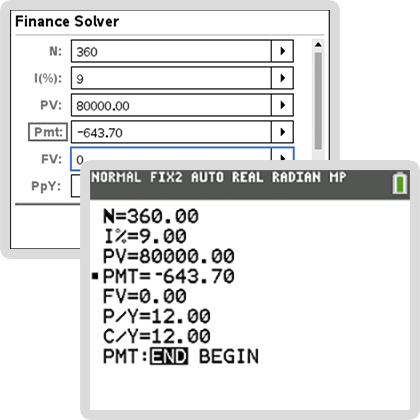## Amortization Tables

Prepare amortization tables indicating principal payments, interest payments and the balance on a loan for each time interval.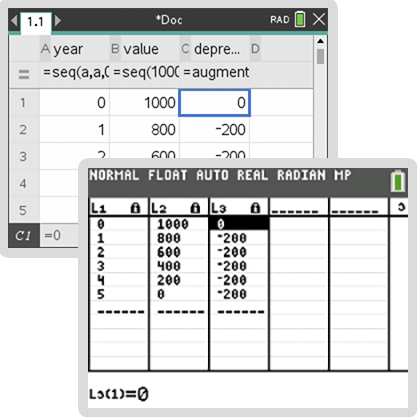## Depreciation

Explore the depreciation of assets and methods such as straight-line depreciation, sum-of-the-digits method and double-declining balance depreciation.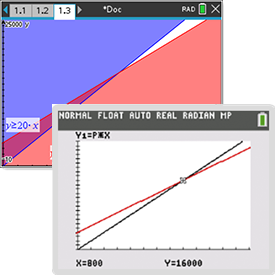## Breakeven Analysis

Perform breakeven analyses using the cost and revenue of an enterprise and determining the relationship between fixed and variable costs, profits, pricing policy and the volume of output.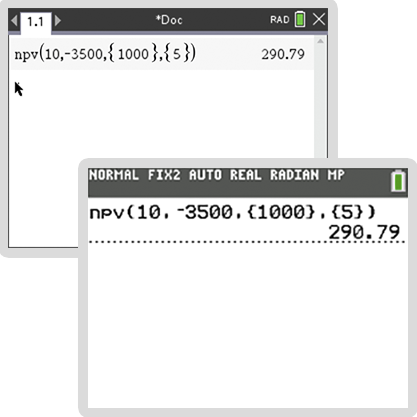## Net Present Value and Internal Rate of Return

Understand cash flow and its present value, comparing alternative investments by determining their net present value and internal rate of return, and choosing whether to lease or borrow money.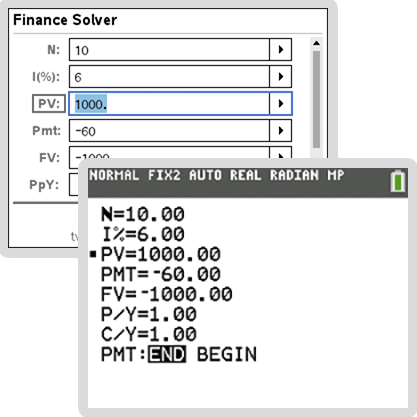## Bonds and Bond Yield to Maturity

In this activity, students estimate the market value of specific bonds and explore bond values versus current market interest rates.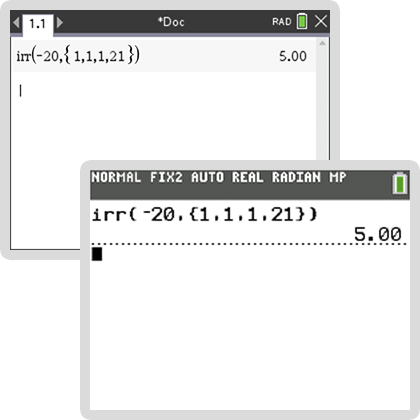## Returns on a Share of Stock

Determine the internal rate of return of cashflows associated with stock purchases, dividends and sales, as well as the impact of varying selling prices.

« BackNext »